@Team 2018-07-20T15:52:16.000000Z 字数 9702 阅读 2001

# 干货|（DL~3）deep learning中一些层的介绍

石文华

# 一、relu层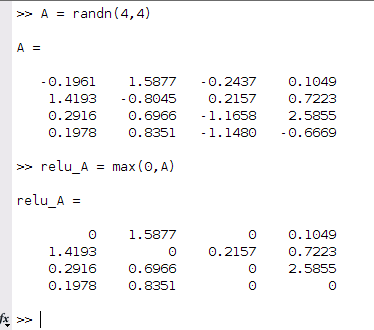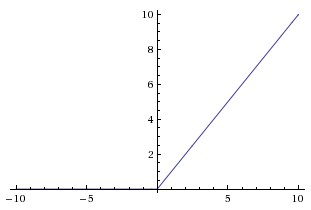relu函数作为激活函数，具有以下功能：
1、易于计算（前向/反向传播），采用sigmoid函数作为激活函数时候（指数运算），计算量大，反向传播求误差梯度时，求导涉及除法，计算量相当大，而采用Relu激活函数，整个过程的计算量节省很多。
2、深度模型中受消失梯度的影响要小得多，对于深层网络，sigmoid函数反向传播时，很容易就出现梯度消失的情况（在sigmoid函数接近饱和区时，变换太缓慢，导数趋于0，这种情况会造成信息丢失），从而无法完成深层网络的训练。
3、如果你使用大的学习率，他们可能会不可逆转地死去，因为当一个非常大的梯度流过一个 ReLU 神经元，更新过参数之后，这个神经元再也不会对任何数据有激活现象了。这个神经元的梯度将一直都是0了。

## 1、前向传播

python实现relu的前向传播：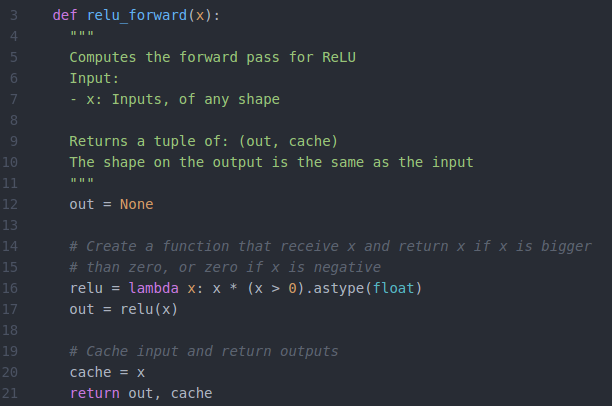## 2、反向传播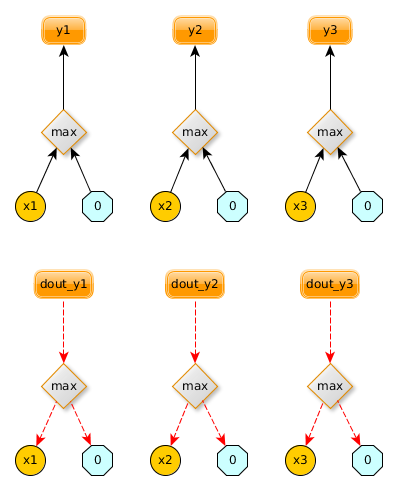python实现relu 反向传播：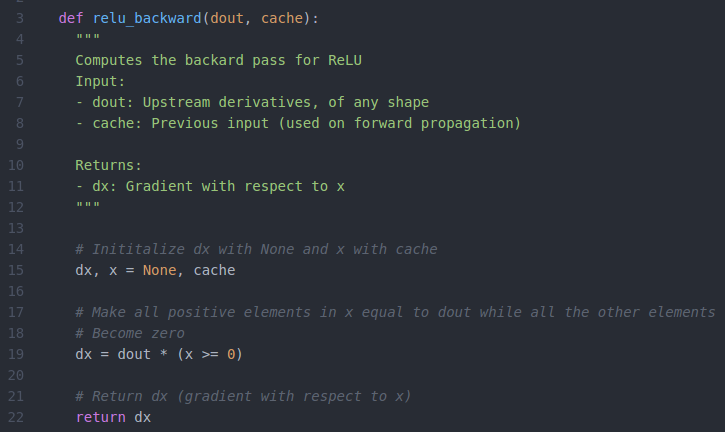# 二、dropout层

Dropout是一种用于防止神经网络过度拟合的技术，你还可以使用L2正则化防止过拟合。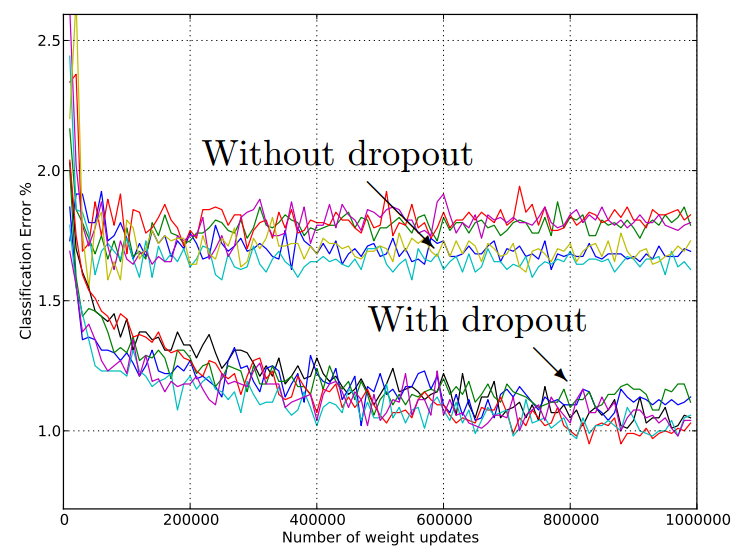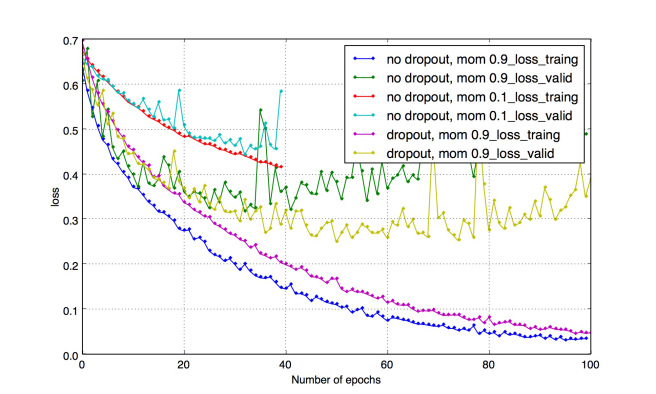## 1、dropout工作原理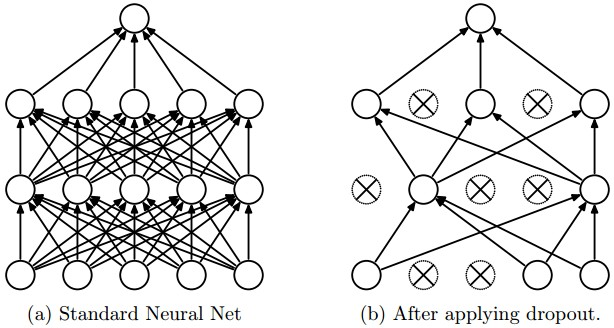## 4、dropout的功效

Dropout背后理念和集成模型很相似。在Drpout层，不同的神经元组合被关闭，这代表了一种不同的结构，所有这些不同的结构使用一个的子数据集并行地带权重训练，而权重总和为1。

Dropout有效的另外一个视点是：由于神经元是随机选择的，所以可以减少神经元之间的相互依赖，从而确保提取出相互独立的重要特征。

## 5、python实现dropout的前向传播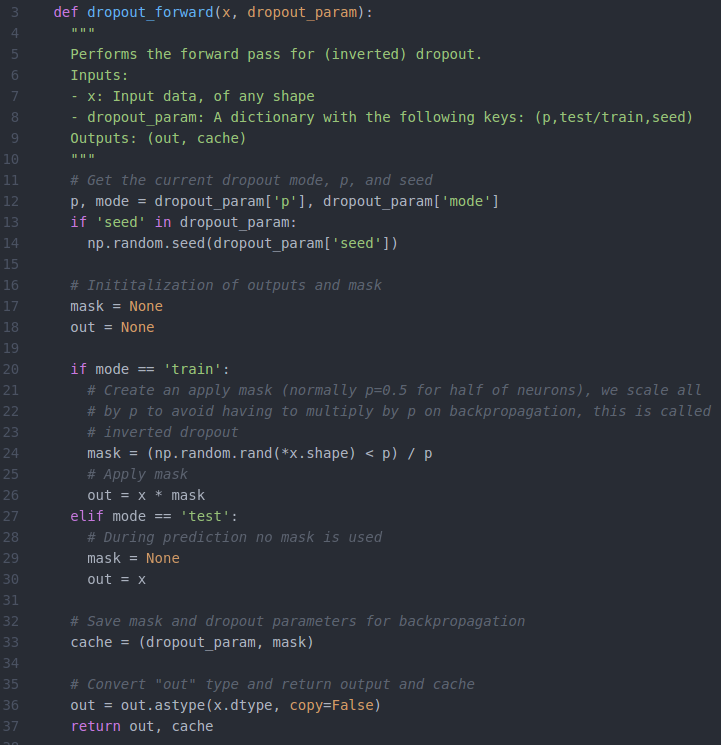## 6、python实现dropout的反向传播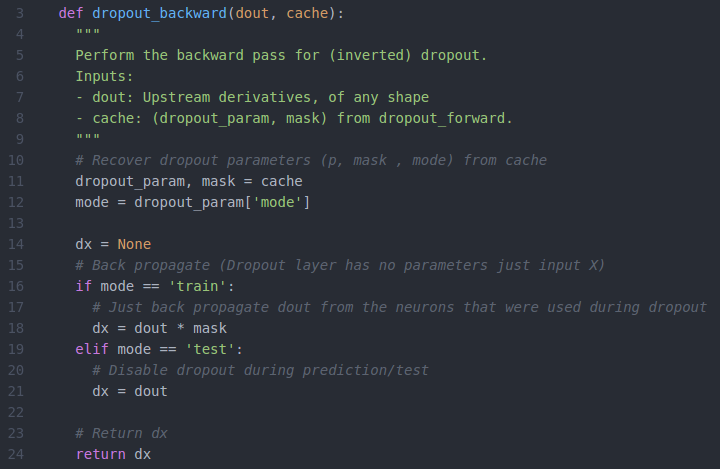# 三、卷积层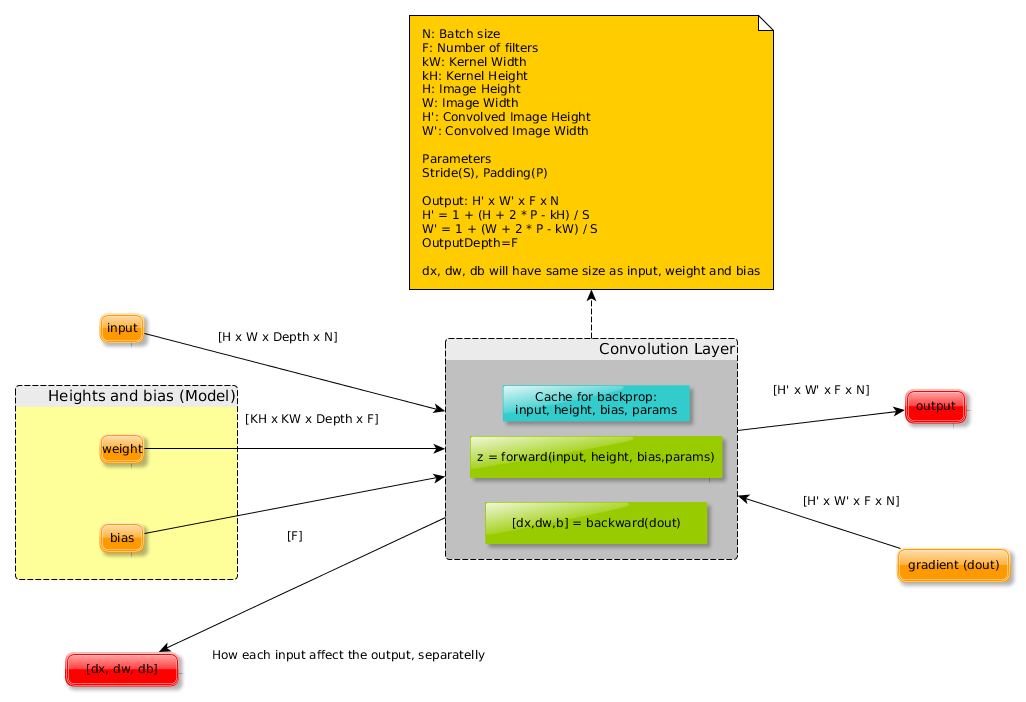N:批处理大小(4d张量上的图像数)
F:卷积层上的滤波器个数
kW/kH:内核宽度/高度(通常我们使用方形卷积核，kW=kH)
H/W:图像高度/宽度(通常H=W)
H'/W':卷积图像高度/宽度(如果使用适当的填充，则与输入相同)
Stride:卷积滑动窗口将要移动的像素数。
Depth:输入特征图的深度(如输入为RGB图像则深度为3)
Output depth:输出的特征图的深度(与F相同)

## 1、前向传播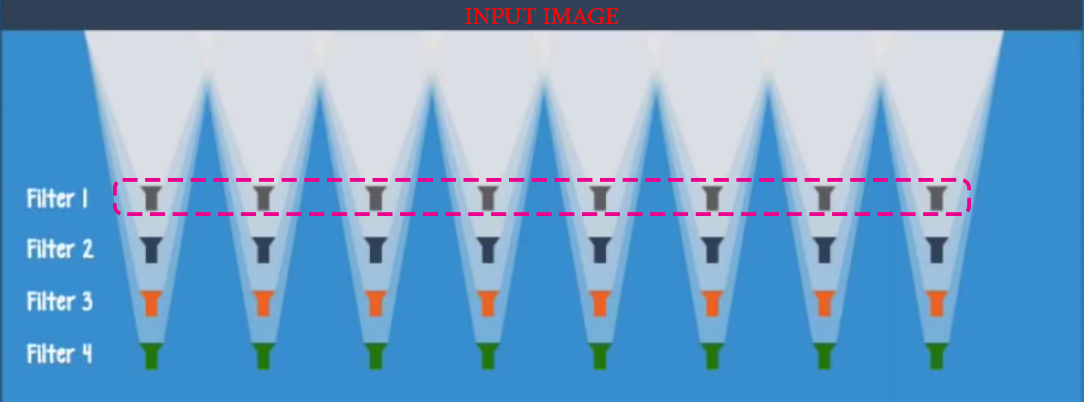## 2、python实现卷积层的前向传播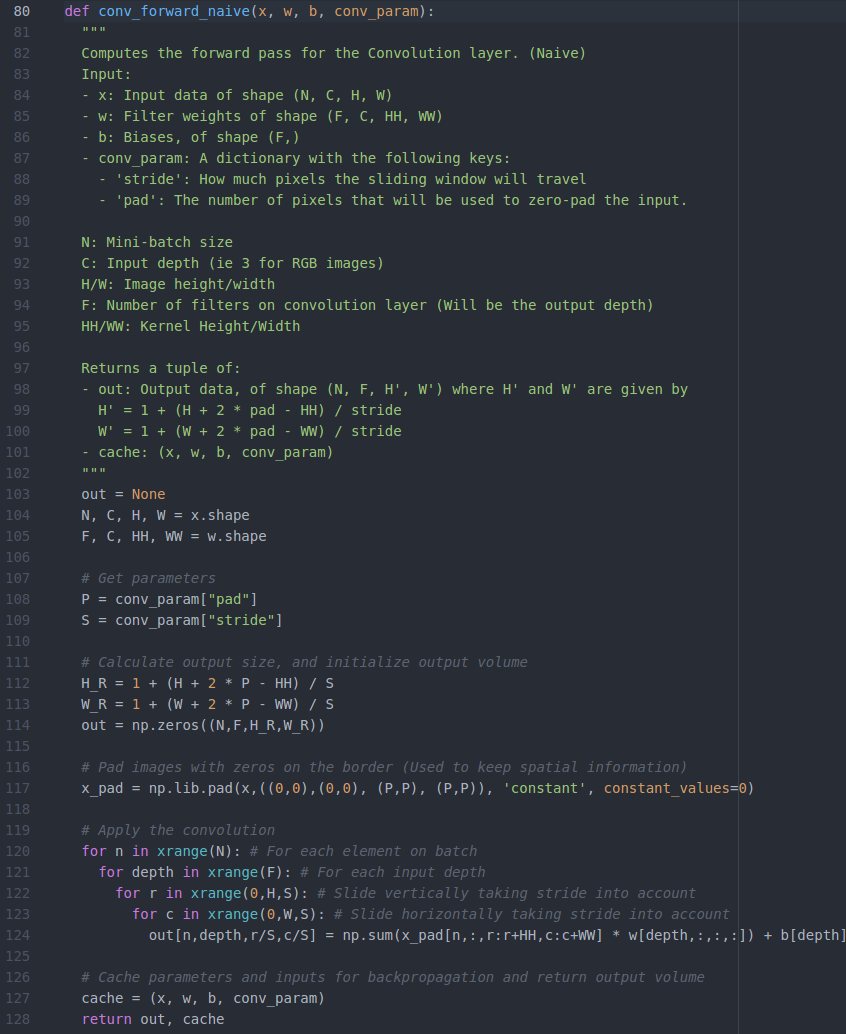## 3、反向传播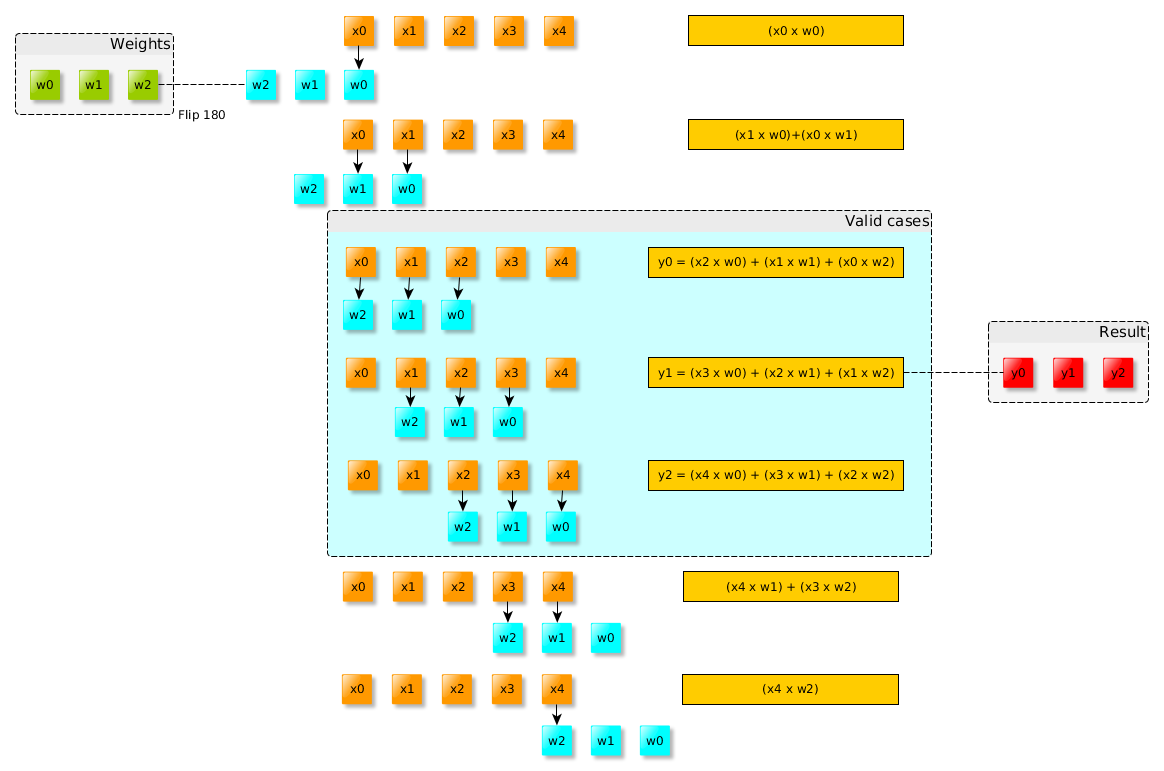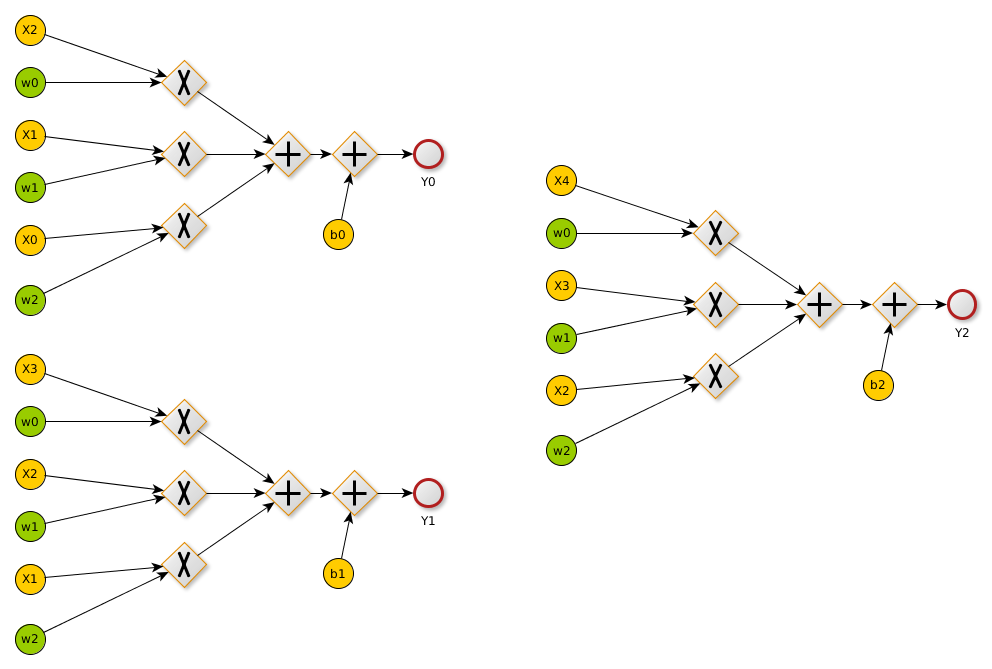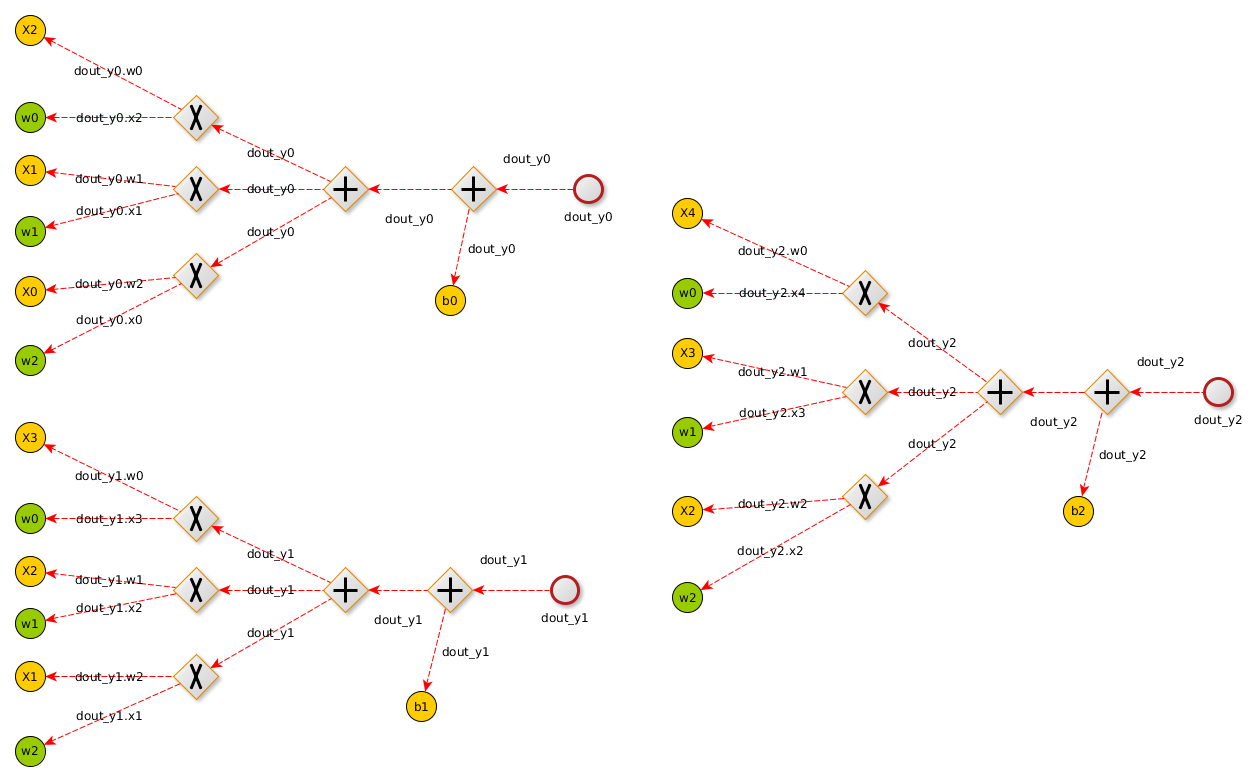dX跟X大小相同，所以我们需要进行填充
dout跟Y大小相同，在本例中为3（渐变输入）

1d卷积的输出尺寸计算公式：outputSize=(InputSize-KernelSize+2P)+1，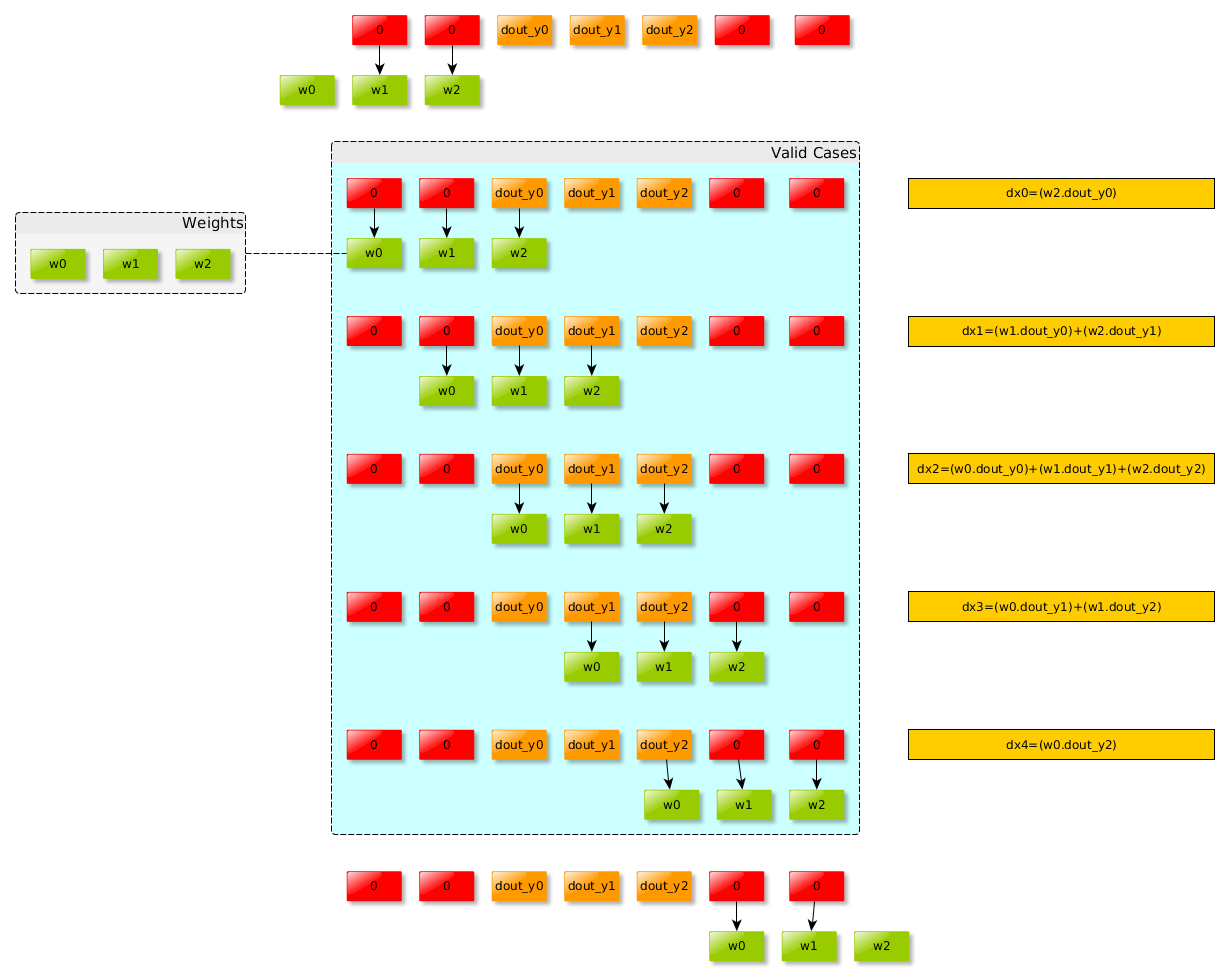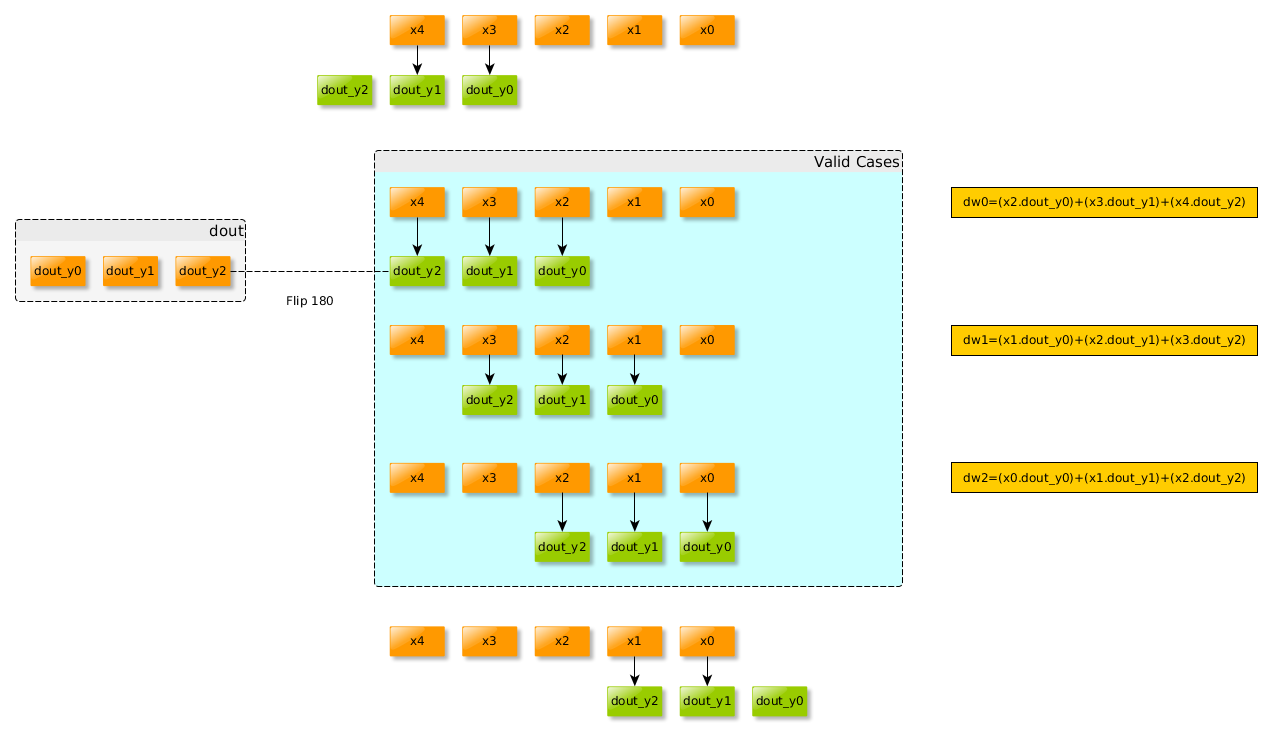## 4、python实现卷积的反向传播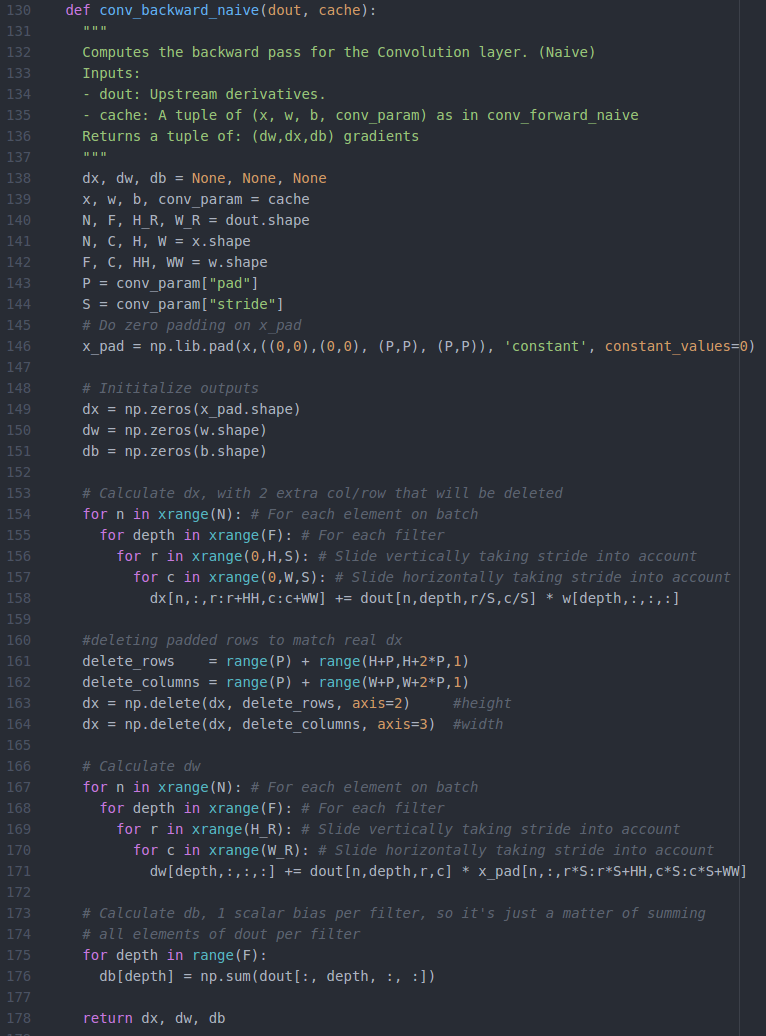## 5、卷积运算转换为矩阵运算

### 5.1 Im2col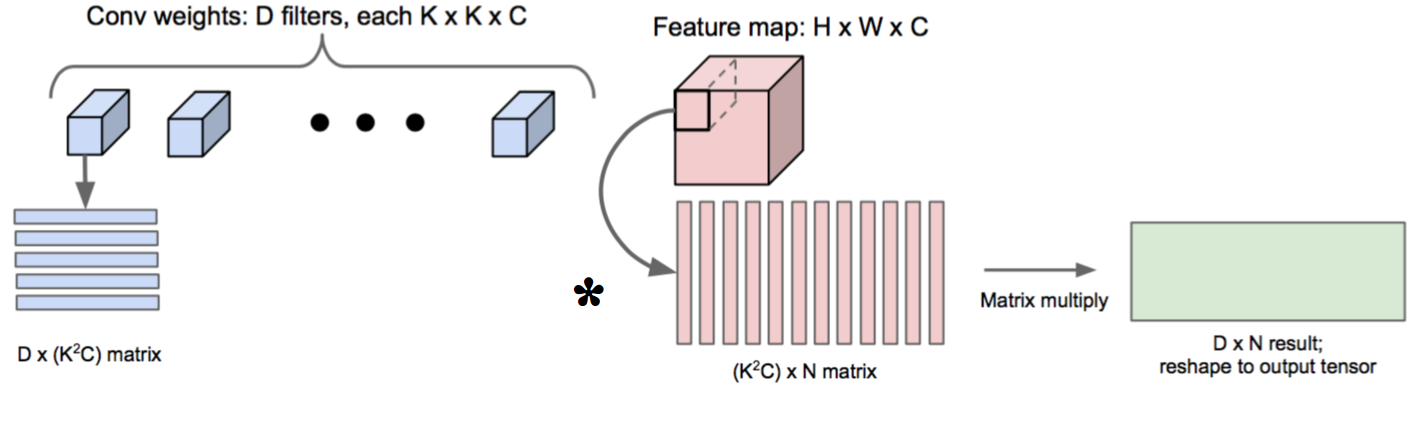[img_height, img_width, img_channels] = size(img);
newImgHeight = floor(((img_height + 2*P - ksize) / S)+1);
newImgWidth = floor(((img_width + 2*P - ksize) / S)+1);
cols = single(zeros((img_channels*ksize*ksize),(newImgHeight * newImgWidth)));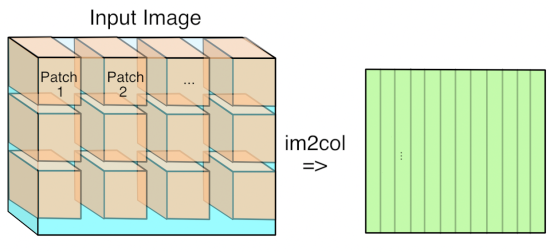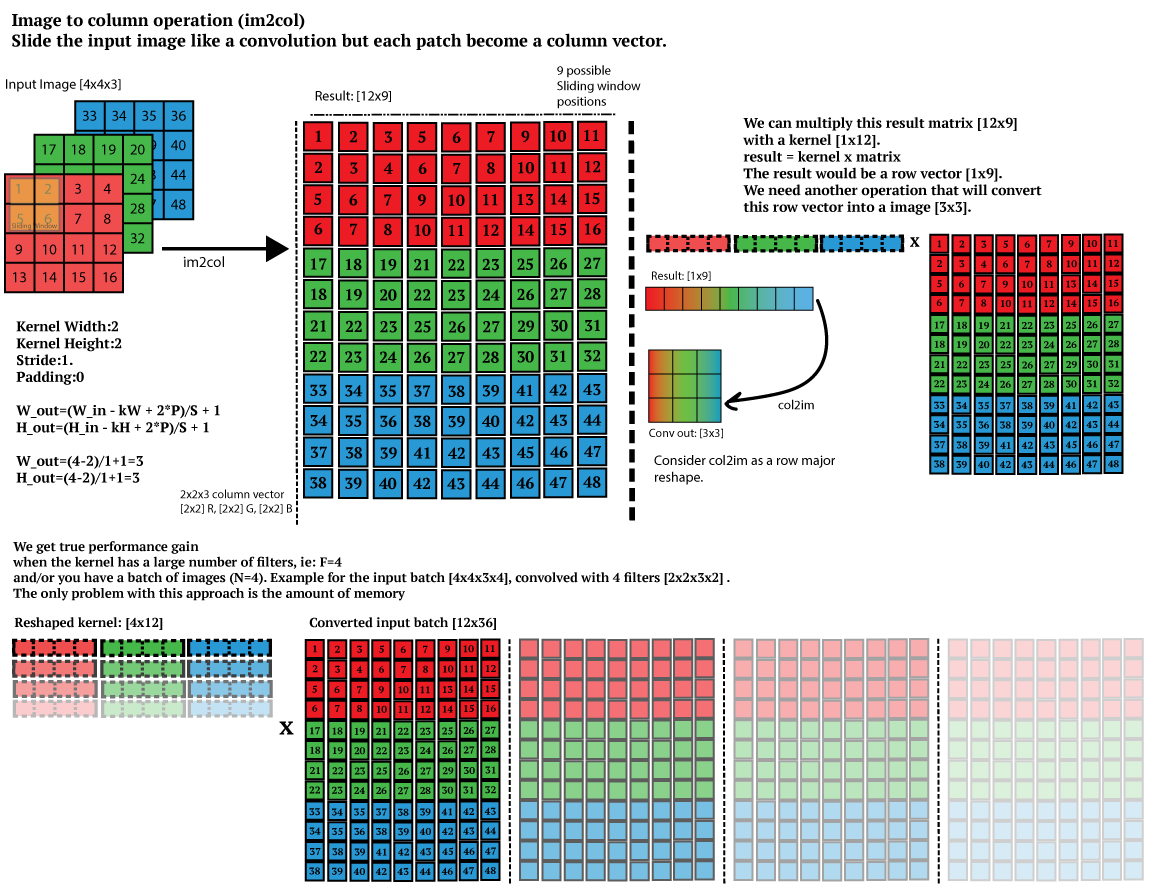### 5.2前向传播计算图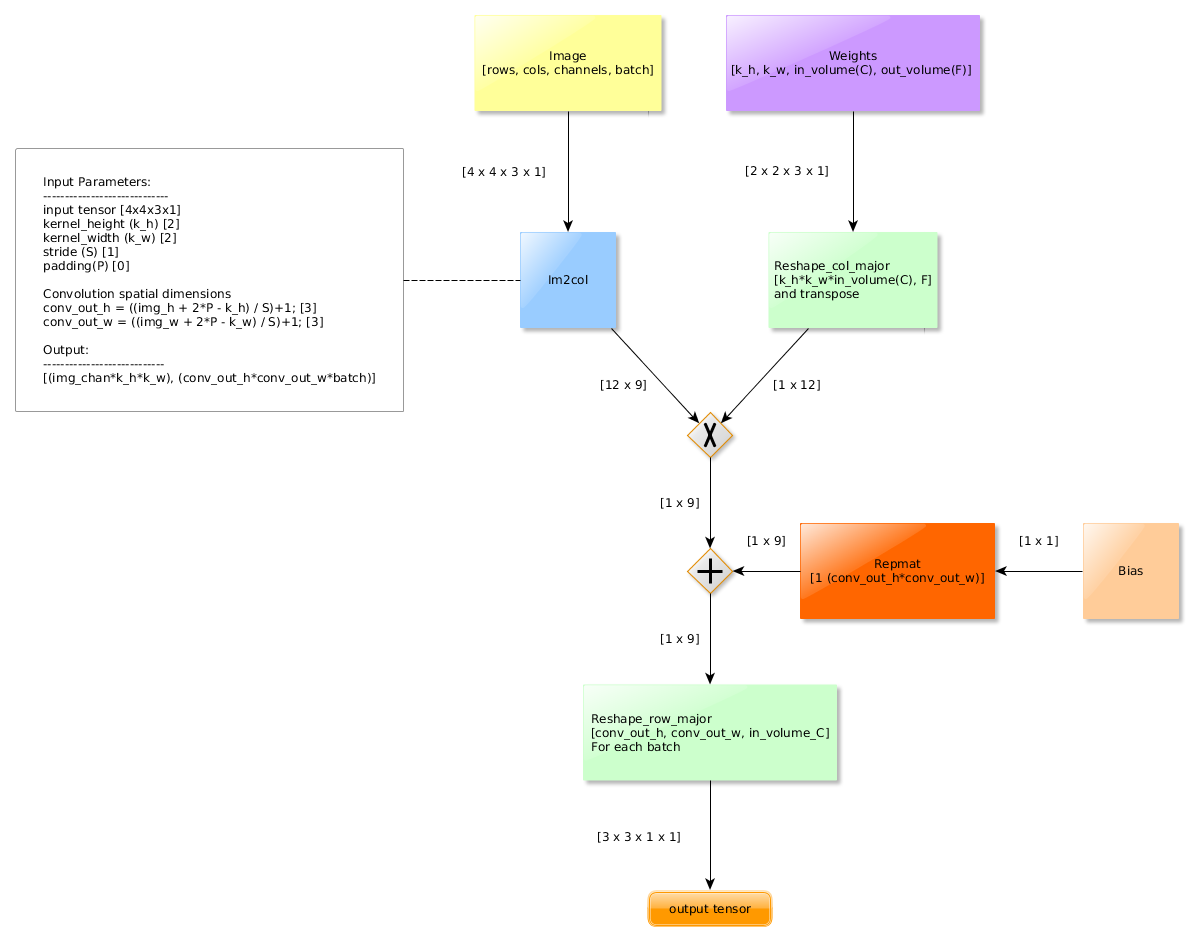def conv_forward_naive(x, w, b, conv_param):  """  A naive implementation of the forward pass for a convolutional layer.  The input consists of N data points, each with C channels, height H and width  W. We convolve each input with F different filters, where each filter spans  all C channels and has height HH and width HH.  Input:  - x: Input data of shape (N, C, H, W)  - w: Filter weights of shape (F, C, HH, WW)  - b: Biases, of shape (F,)  - conv_param: A dictionary with the following keys:    - 'stride': The number of pixels between adjacent receptive fields in the      horizontal and vertical directions.    - 'pad': The number of pixels that will be used to zero-pad the input.  Returns a tuple of:  - out: Output data, of shape (N, F, H', W') where H' and W' are given by    H' = 1 + (H + 2 * pad - HH) / stride    W' = 1 + (W + 2 * pad - WW) / stride  - cache: (x, w, b, conv_param)  """  out = None  pad_num = conv_param['pad']  stride = conv_param['stride']  N,C,H,W = x.shape  F,C,HH,WW = w.shape  H_prime = (H+2*pad_num-HH) // stride + 1  W_prime = (W+2*pad_num-WW) // stride + 1  out = np.zeros([N,F,H_prime,W_prime])  #im2col  for im_num in range(N):      im = x[im_num,:,:,:]      im_pad = np.pad(im,((0,0),(pad_num,pad_num),(pad_num,pad_num)),'constant')      im_col = im2col(im_pad,HH,WW,stride)      filter_col = np.reshape(w,(F,-1))      mul = im_col.dot(filter_col.T) + b      out[im_num,:,:,:] = col2im(mul,H_prime,W_prime,1)  cache = (x, w, b, conv_param)  return out, cache

im2col函数：

def im2col(x,hh,ww,stride):    """    Args:      x: image matrix to be translated into columns, (C,H,W)      hh: filter height      ww: filter width      stride: stride    Returns:      col: (new_h*new_w,hh*ww*C) matrix, each column is a cube that will convolve with a filter            new_h = (H-hh) // stride + 1, new_w = (W-ww) // stride + 1    """    c,h,w = x.shape    new_h = (h-hh) // stride + 1    new_w = (w-ww) // stride + 1    col = np.zeros([new_h*new_w,c*hh*ww])    for i in range(new_h):       for j in range(new_w):           patch = x[...,i*stride:i*stride+hh,j*stride:j*stride+ww]           col[i*new_w+j,:] = np.reshape(patch,-1)    return col

### 5.3反向传播图

，不同之处在于有一堆重塑，转置和im2col块。

im2col反向传播操作时。无法实现简单的重塑。这是因为感受野实际上是重合的（取决于步长），所以需要将感受野相交的地方的梯度相加。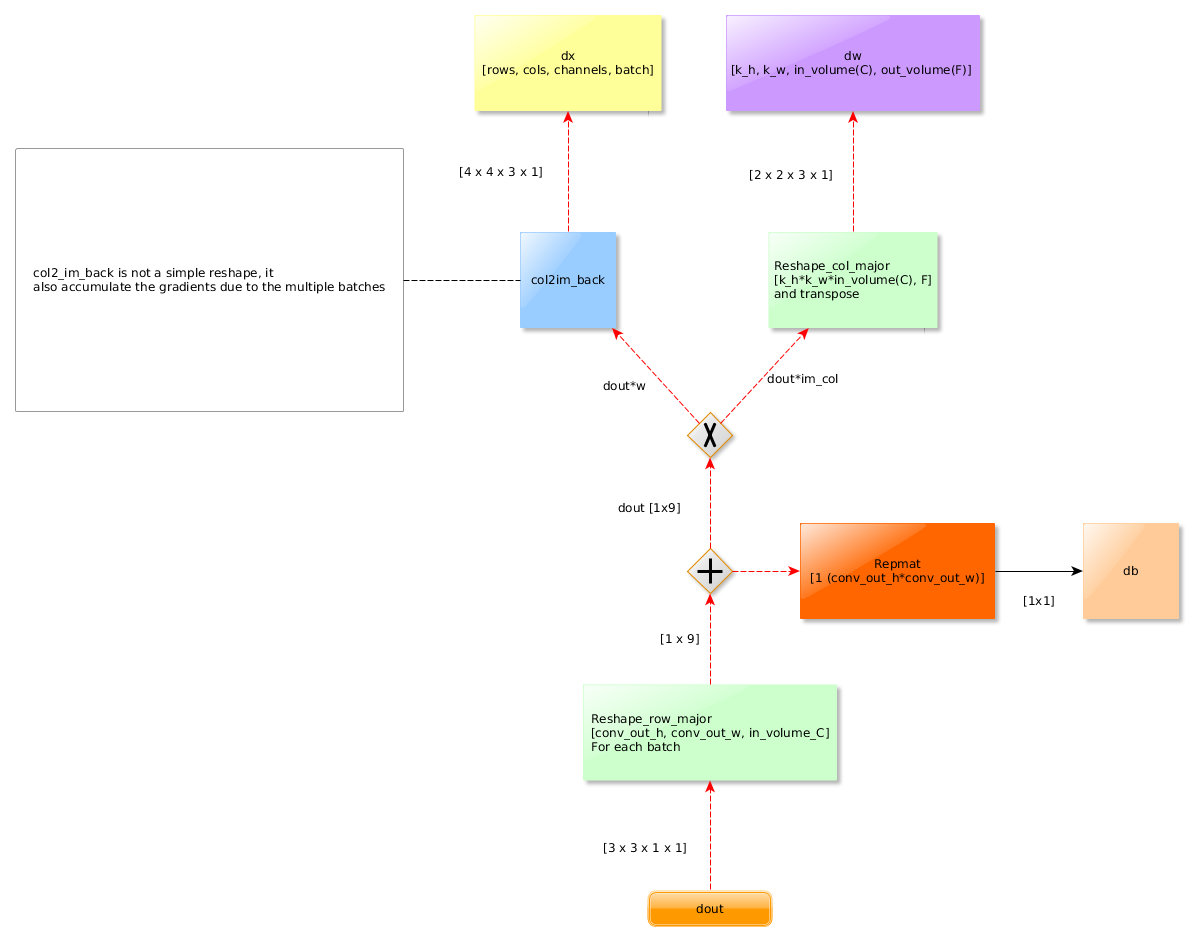def conv_backward_naive(dout, cache):  """  A naive implementation of the backward pass for a convolutional layer.  Inputs:  - dout: Upstream derivatives.  - cache: A tuple of (x, w, b, conv_param) as in conv_forward_naive  Returns a tuple of:  - dx: Gradient with respect to x  - dw: Gradient with respect to w  - db: Gradient with respect to b  """  dx, dw, db = None, None, None  x, w, b, conv_param = cache  pad_num = conv_param['pad']  stride = conv_param['stride']  N,C,H,W = x.shape  F,C,HH,WW = w.shape  H_prime = (H+2*pad_num-HH) // stride + 1  W_prime = (W+2*pad_num-WW) // stride + 1  dw = np.zeros(w.shape)  dx = np.zeros(x.shape)  db = np.zeros(b.shape)  # We could calculate the bias by just summing over the right dimensions  # Bias gradient (Sum on dout dimensions (batch, rows, cols)  #db = np.sum(dout, axis=(0, 2, 3))  for i in range(N):      im = x[i,:,:,:]      im_pad = np.pad(im,((0,0),(pad_num,pad_num),(pad_num,pad_num)),'constant')      im_col = im2col(im_pad,HH,WW,stride)      filter_col = np.reshape(w,(F,-1)).T      dout_i = dout[i,:,:,:]      dbias_sum = np.reshape(dout_i,(F,-1))      dbias_sum = dbias_sum.T      #bias_sum = mul + b      db += np.sum(dbias_sum,axis=0)      dmul = dbias_sum      #mul = im_col * filter_col      dfilter_col = (im_col.T).dot(dmul)      dim_col = dmul.dot(filter_col.T)      dx_padded = col2im_back(dim_col,H_prime,W_prime,stride,HH,WW,C)      dx[i,:,:,:] = dx_padded[:,pad_num:H+pad_num,pad_num:W+pad_num]      dw += np.reshape(dfilter_col.T,(F,C,HH,WW))  return dx, dw, db

col2im函数：

def col2im(mul,h_prime,w_prime,C):    """      Args:      mul: (h_prime*w_prime*w,F) matrix, each col should be reshaped to C*h_prime*w_prime when C>0, or h_prime*w_prime when C = 0      h_prime: reshaped filter height      w_prime: reshaped filter width      C: reshaped filter channel, if 0, reshape the filter to 2D, Otherwise reshape it to 3D    Returns:      if C == 0: (F,h_prime,w_prime) matrix      Otherwise: (F,C,h_prime,w_prime) matrix    """    F = mul.shape    if(C == 1):        out = np.zeros([F,h_prime,w_prime])        for i in range(F):            col = mul[:,i]            out[i,:,:] = np.reshape(col,(h_prime,w_prime))    else:        out = np.zeros([F,C,h_prime,w_prime])        for i in range(F):            col = mul[:,i]            out[i,:,:] = np.reshape(col,(C,h_prime,w_prime))    return out

col2im_back函数：

def col2im_back(dim_col,h_prime,w_prime,stride,hh,ww,c):    """    Args:      dim_col: gradients for im_col,(h_prime*w_prime,hh*ww*c)      h_prime,w_prime: height and width for the feature map      strid: stride      hh,ww,c: size of the filters    Returns:      dx: Gradients for x, (C,H,W)    """    H = (h_prime - 1) * stride + hh    W = (w_prime - 1) * stride + ww    dx = np.zeros([c,H,W])    for i in range(h_prime*w_prime):        row = dim_col[i,:]        h_start = (i / w_prime) * stride        w_start = (i % w_prime) * stride        dx[:,h_start:h_start+hh,w_start:w_start+ww] += np.reshape(row,(c,hh,ww))    return dx

### 5.4小案例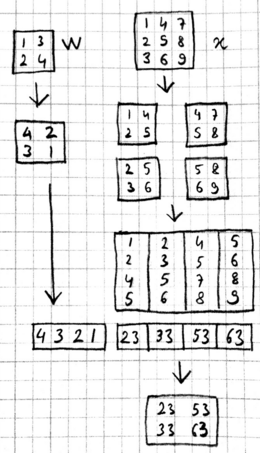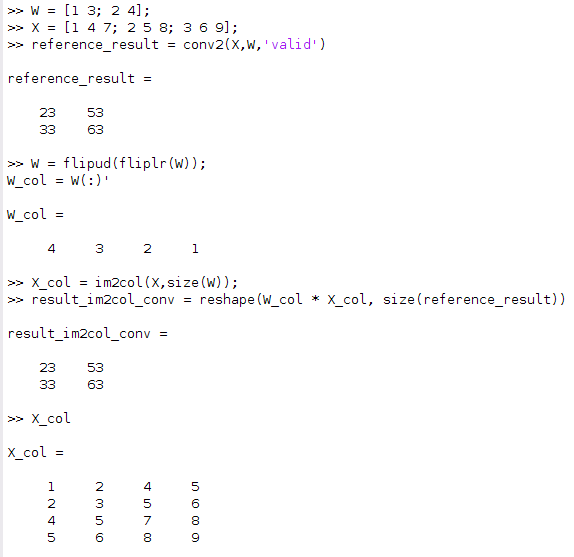# 四、池化层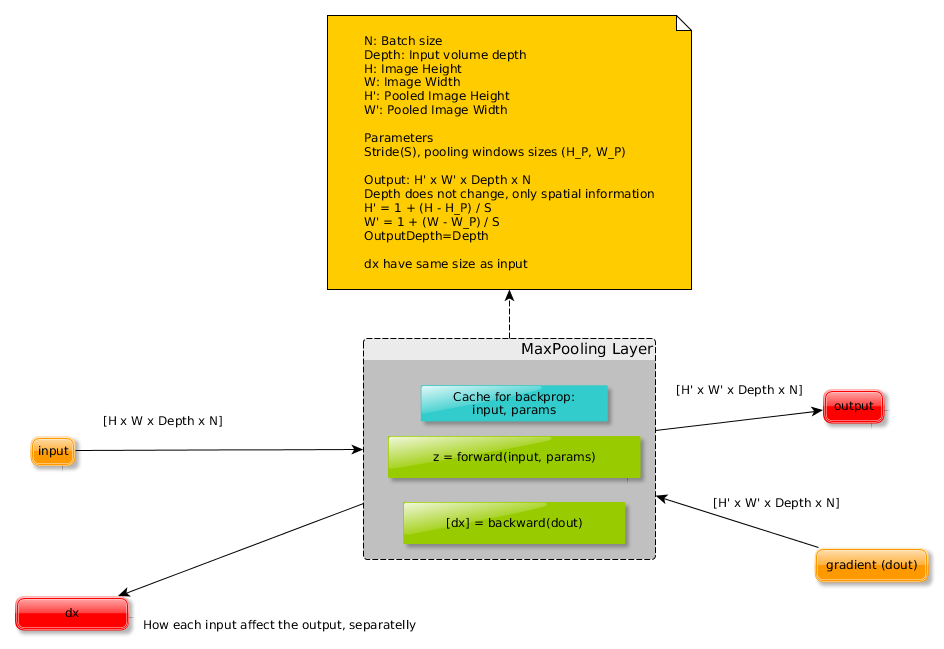1、减少了特征空间信息，内存的使用更少，计算速度也将更快
2、防止过拟合
3、引入了位移不变性，更关注是否存在某些特征而不是特征具体的位置。比如最常见的max pooling，因为取一片区域的最大值，所以这个最大值在该区域内无论在哪，max-pooling之后都是它，相当于对微小位移的不变性。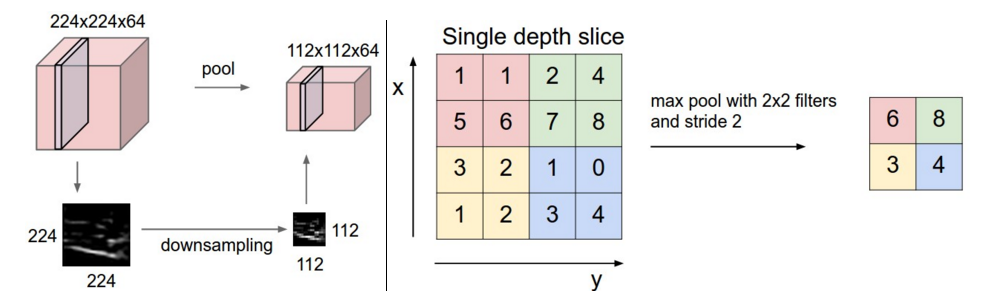1、输入：H1 x W1 x Depth_In x N.
2、步长：控制窗口滑动的像素数量的标量。
3、K：内核大小
3、输出：H2 x W2 x Depth_Out x N: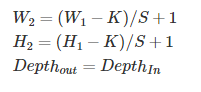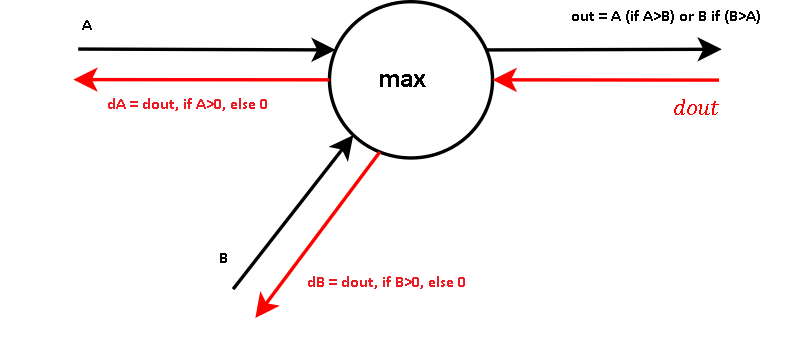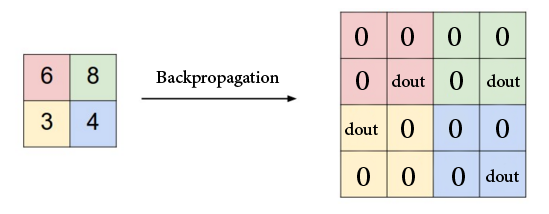## 1、python实现池化层的前向传播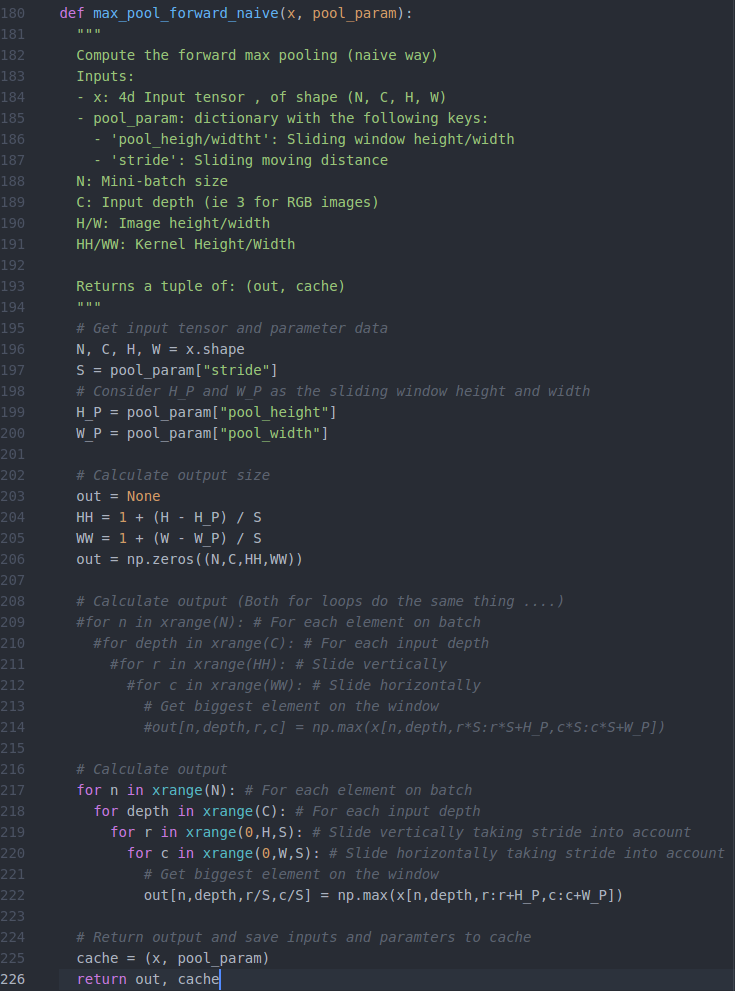## 2、python实现池化层的反向传播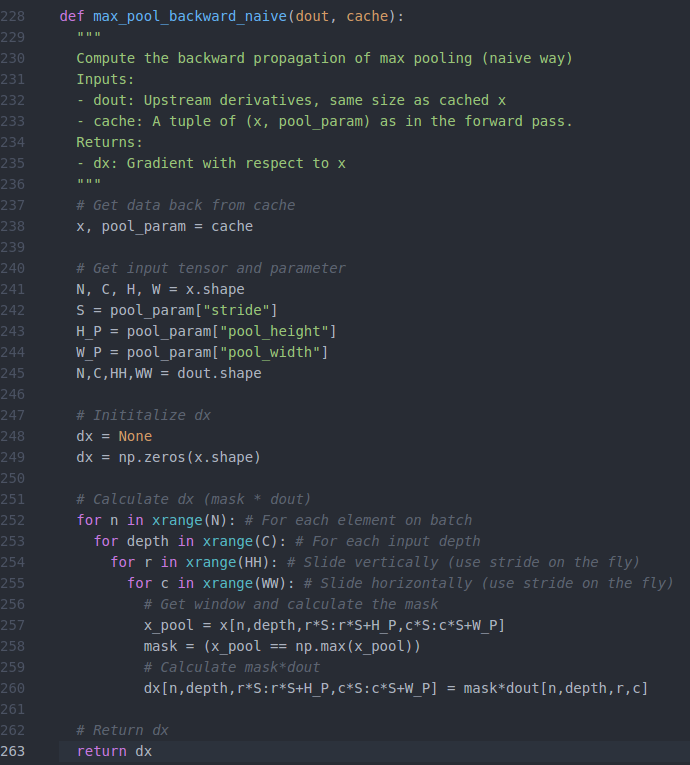• 私有
• 公开
• 删除